• 2022-03-31 13:33:38

1、导包

import numpy as np
import pandas as pd
import matplotlib.pyplot as plt
from sklearn.linear_model import LinearRegression
plt.rcParams["font.sans-serif"] = ['SimHei']

2、局部加权线性回归

# 局部加权线性回归
# 增加了核函数，使用高斯核函数，相当于只用于当前数据点相近的部分数据计算回归系数
# x训练集的特征矩阵，y训练集的标签矩阵
def lrlw(test_point, x, y, k):
xMat = np.mat(x)  #将数据转换为矩阵
yMat = np.mat(y).T
m = np.shape(x)  #数据总行数
weights = np.mat(np.eye(m))  # 生成对角矩阵
for i in range(m):
diffMat = test_point - xMat[:, i]  # 计算与数据集中其他点的距离
weights[i, i] = np.exp(diffMat * diffMat.T / (-2.0 * k ** 2))  #计算权重对角矩阵
xtx = (xMat * weights) * xMat.T  # 对x值进行加权计算

if np.linalg.det(xtx) == 0:
print("the matrix is singular, cannot do inverse")
return 0
y = xtx.I * xMat * weights * yMat  # 计算回归系数对y加权
return test_point * y

# 对所有点计算估计值
def lrlwTest(xd, yd, k):
ytest = np.zeros(xd.shape)  # 生成一个为0的一维向量
for i in range(xd.shape):
ytest[i] = lrlw(xd[i], xd, yd, k)
return ytest

def lrlw_display(xd, yd, K):
ytest = lrlwTest(xd, yd, K)
# 返回数据从小到大的索引值
sorted_index = xd.argsort(0)
x_sorted = xd[sorted_index]
plt.plot(x_sorted, ytest[sorted_index])  # 折线图
plt.scatter(xd, yd, color='red')
plt.title("局部加权线性回归 K = {}".format(K), fontproperties="SimHei", fontSize=16)
plt.show()

3、岭回归

# 岭回归
# 计算ws (xTx+IMat).I * xTy
def ridgeRegres(testPoint, xMat, yMat, lam):
xTx = xMat * xMat.T
denom = xTx + np.eye(np.shape(xMat)) * lam
if np.linalg.det(denom) == 0.0:
print("矩阵不可逆")
return
ws = denom.I * (xMat * yMat)
return ws * testPoint

def ridgeTest(xArr, yArr, lam):
yhat = np.zeros(xArr.shape)
for i in range(xArr.shape):  # 测试不同的lambda取值，获得系数
yhat[i] = ridgeRegres(xArr[:, i], xArr, yArr, lam)
return yhat

def ridge_display(xd, yd, lam):
xmat = np.mat(xd)
ymat = np.mat(yd).T
ridge = ridgeTest(xmat, ymat, lam)
sorted_index = xd.argsort(0)
x_sorted = xd[sorted_index]
plt.plot(x_sorted, ridge[sorted_index])
plt.title("岭回归 lam = {}".format(lam), fontproperties="SimHei", fontSize=16)
plt.scatter(xd, yd, color='red')
plt.show()


4、LASSO回归

def LASSO(xd, yd, lam, learning_rate=0.01, epochs=2000):
# 归一化
x_normal = (xd - xd.mean()) / xd.std()
x_raw = x_normal.reshape(-1, 1)
y_raw = yd.reshape(-1, 1)
w = np.random.randn(x_raw.shape, 1)
b = 0
for i in range(epochs):
num_train = x_raw.shape
y_hat = np.dot(x_raw, w) + b
w -= learning_rate * (np.dot(x_raw.T, (y_hat - y_raw)) / num_train + lam)
b -= learning_rate * (np.sum((y_hat - y_raw)) / num_train)
plt.plot(xd, w * x_raw + b)
plt.title("LASSO lam = {}".format(lam), fontproperties="SimHei", fontSize=16)
plt.scatter(xd, yd, color='green')
plt.show()


5、主函数

if __name__ == "__main__":
data = data.values
xd = data[:, 0]  # 取二维数组的第一维的所有数据，取第二维的第一位数据
yd = data[:, 1]
lrlw_display(xd, yd, 0.01)
lrlw_display(xd, yd, 0.05)
lrlw_display(xd, yd, 0.1)
lrlw_display(xd, yd, 1)
ridge_display(xd, yd, 3)
ridge_display(xd, yd, 0.1)
LASSO(xd, yd, 0.15)
LASSO(xd, yd, 0.33)

6、数据集
链接：https://pan.baidu.com/s/1JyaeeAPRhkYsN7DGzsBuWQ?pwd=4S6g
提取码：4S6gpython 机器学习
更多相关内容
• 利用 python 进行局部加权线性回归实战 ，内含有原始数据及拟合结论图。
• 局部加权线性回归模型2. 求解方法3. 代码实现 前言 ​ 线性回归只能拟合线性曲面（广义的曲面），如果一个回归任务中的输出变量 y  (y∈R)y\,\,\left( y\in \mathbb{R} \right)y(y∈R) 关于特征向量 x=(x0,x1,...

# 前言

​ 线性回归只能拟合线性曲面（广义的曲面），如果一个回归任务中的输出变量 y    ( y ∈ R ) y\,\,\left( y\in \mathbb{R} \right) 关于特征向量 x = ( x 0 , x 1 , ⋯   , x n )    ( x ∈ R n + 1 , x 0 = 1 ) x=\left( x_0,x_1,\cdots ,x_n \right) \,\,\left( x\in \mathbb{R} ^{n+1},x_0=1 \right) 不是线性的，那怎样拟合可以比较好呢？局部加权线性回归是一种方法。

# 1. 局部加权线性回归模型

​ 当两个样本点的特征向量距离较近时，它们的输出变量通常比较接近，也就是说它们具有相似的性质。现在我们有 m m 个训练样本点 ( x ( 1 ) , y ( 1 ) ) , ( x ( 2 ) , y ( 2 ) ) , ⋯   , ( x ( m ) , y ( m ) ) \left( x^{\left( 1 \right)},y^{\left( 1 \right)} \right) ,\left( x^{\left( 2 \right)},y^{\left( 2 \right)} \right) ,\cdots ,\left( x^{\left( m \right)},y^{\left( m \right)} \right) 构成训练集。那么当我们给一个特征向量 x x 时，要根据训练集中的信息预测出它的输出变量 y y ，距离 x x 越近的训练集中的样本点越应该被重视。换句话说，我们需要给训练集中的每个样本点一个权值，距离 x x 越近的样本点的权值应该越大，这是赋权的一个原则，那么具体应该如何赋权呢？

​ 首先应该想一想，我们怎样衡量距离呢？欧氏距离（2范数）是一种常用的衡量方式，即：
d i s t ( x , x ( i ) ) = ∥ x − x ( i ) ∥ 2 dist\left( x,x^{\left( i \right)} \right) =\left\| x-x^{\left( i \right)} \right\| _2
我们用下面的公式给训练集中第 i i 样本点赋权值：
w i = exp ⁡ ( − ∥ x − x ( i ) ∥ 2 2 2 σ 2 ) w_i=\exp \left( -\frac{\left\| x-x^{\left( i \right)} \right\| _{2}^{2}}{2\sigma ^2} \right)
这里似乎很突兀，还不知道为什么，直接就给出了这样的公式，我也不太清楚为什么，这个算法经常使用这样的函数来赋权，对于不是很想深入理论研究的我姑且认为是玄学吧！当然肯定不是玄学，这样做应该是有一定理论依据的，不过我就暂时不了解了。

​ 对于给定的特征向量作为输入，我们要预测它的输出变量，使用下面的经过加权处理后的损失函数：
J ( θ ) = 1 2 ∑ i = 1 m w i ( h θ ( x ( i ) ) − y ( i ) ) 2 J\left( \theta \right) =\frac{1}{2}\sum_{i=1}^m{w_i\left( h_{\theta}\left( x^{\left( i \right)} \right) -y^{\left( i \right)} \right) ^2}
这里还是挺自然的，就是一个简单的加权求和，最小化这样的损失函数时，算法肯定会寻找使得距离 x x 越近的样本点的损失越小 θ \theta ，也就是说算法更加重视了那些距离 x x 近的点，这也有利于更准确地预测 y y 。该函数使用矩阵的方式写出来就是：
J ( θ ) = 1 2 ( X θ − y ) T W ( X θ − y ) J\left( \theta \right) =\frac{1}{2}\left( X\theta -y \right) ^TW\left( X\theta -y \right)
其中权值矩阵 W = d i a g ( w 1 , w 2 , ⋯   , w m ) W=\mathrm{diag}\left( w_1,w_2,\cdots ,w_m \right)

​ 优化这个损失函数的过程就是该算法的训练过程。优化这个损失函数后，就可以使用得到参数 θ \theta ，进而使用 h θ ( x ) h_{\theta}\left( x \right) 预测输出变量 y y 。 如果我们有 K K 个特征向量需要被预测，记这 K K 个特征向量为 x ( m + 1 ) , x ( m + 2 ) , ⋯   , x ( m + K ) x^{\left( m+1 \right)},x^{\left( m+2 \right)},\cdots ,x^{\left( m+K \right)} ，那么对于每一个特征向量 x ( m + i )    ( 1 ⩽ i ⩽ K ) x^{\left( m+i \right)}\,\,\left( 1\leqslant i\leqslant K \right) 我们都要计算一次权值矩阵，然后然后优化损失函数得到 θ \theta ，进而预测输出变量 y ( m + i ) y^{\left( m+i \right)}

# 2. 求解方法

​ 梯度下降法是一种很常用的优化方法，这里当然也可以使用梯度下降法，主要就是求解出损失函数的梯度，求解梯度实际上也就是求导数，对其求导：
∂ J ( θ ) ∂ θ = X T W ( X θ − y ) = X T W X θ − X T W y \frac{\partial J\left( \theta \right)}{\partial \theta}=X^TW\left( X\theta -y \right) =X^TWX\theta -X^TWy
这样也就可以使用梯度下降法了，这里就不多说了，直接看正规方程的方法，令其导数为 0 0 可以解出该函数的极小值点：
θ = ( X T W X ) − 1 X T W y \theta =\left( X^TWX \right) ^{-1}X^TWy
​ 值得注意的是，局部加权线性回归时间代价十分高，因为对于每一个需要预测的点，我们都要求出相对应的权值矩阵并且单独训练。而普通的线性回归算法只需要训练一次即可预测所有的点。

​ 局部加权线性回归的好处就是它几乎可以拟合任意形状，只要超参数 σ \sigma 选取合适，那么该算法可以拟合的较好。实际生活中，数据的输出变量和特征向量是线性关系的情况不多，因此纯线性回归模型可能适用性不强，但是局部加权线性回归可以对非线性的数据分布做出较好的拟合。

# 3. 代码实现

​ 写出局部加权线性回归函数。

import numpy as np

def calweight(a, b): # 求解权值的函数
sigma = 0.3
return np.exp(-np.linalg.norm(a-b)**2 / (2*sigma**2))

def LWLR(X_train, y_train, X_test): # 局部加权线性回归
X_train = np.insert(X_train,0,1,axis=1)
X_test = np.insert(X_test, 0, 1, axis = 1)
y_pred = np.empty(X_test.shape) # 预测值

for k in range(X_test.shape): # 对所有的需要预测的特征向量先训练再预测
# 求解权值矩阵
w = np.empty(X_train.shape)
for i in range(X_train.shape):
w[i] = calweight(X_test[k], X_train[i])
W = np.diag(w) #权值矩阵

theta=np.dot(np.linalg.pinv(np.dot(np.dot(X_train.T,W),X_train)),np.dot(np.dot(X_train.T,W),y_train))
y_pred[k] = np.dot(theta,X_test[k]) # 预测

return y_pred


​ 创造数据，使用该函数拟合回归曲线。

import matplotlib.pyplot as plt

def CreateData():
X = np.arange(0,10,0.1)
y = np.empty(X.shape)
for i in range(X.shape):
y[i] = X[i]**3 - 10*X[i]**2 + X[i] + np.random.uniform(-10,10)
return X[:,np.newaxis], y

np.random.seed(0)
X, y = CreateData()
plt.scatter(X, y) # 可视化
X_test = np.arange(0.05,10,0.1) #测试集，目的是通过其画出拟合曲线
X_test = X_test[:,np.newaxis]
y_pred = LWLR(X, y, X_test)
plt.plot(X_test, y_pred, color='red') # 画出拟合曲线
plt.show()


​ 拟合曲线如下：

σ = 2 \sigma=2 ，欠拟合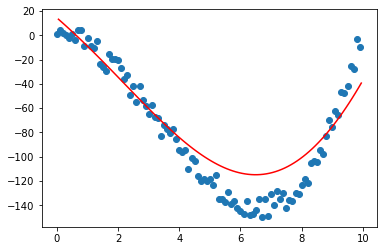σ = 1 \sigma=1 ，感觉在底部稍微有点欠拟合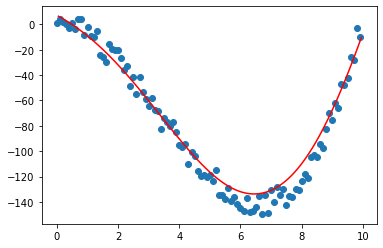σ = 0.5 \sigma=0.5 ，拟合效果不错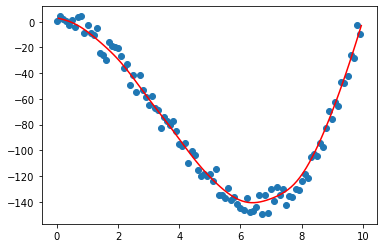σ = 0.1 \sigma=0.1 ，有些过拟合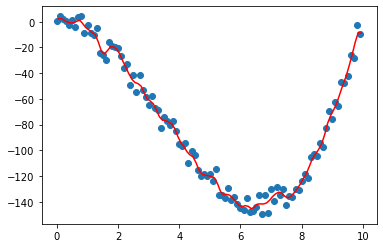​ 只要超参数 σ \sigma 选取合适，很多情况下都会有较好的拟合效果。

展开全文机器学习 人工智能
• 文章目录局部加权线性回归预测鲍鱼年龄 局部加权线性回归 具体理论见上次笔记《线性回归》 预测鲍鱼年龄 import numpy as np class LocalWeightedLinearRegression(object): def __init__(self,train_data,train_...

# 局部加权线性回归

具体理论见上次笔记《线性回归》

## 预测鲍鱼年龄

import numpy as np

class LocalWeightedLinearRegression(object):
def __init__(self,train_data,train_result):
"""
:param train_data: 输入训练数据
:param train_result: 训练数据真实结果
"""
# 获得输入数据集的形状
row, col = np.shape(train_data)
# 构造输入数据数组
self.Train_Data =  * row
# 给每组输入数据增添常数项1
for (index, data) in enumerate(train_data):
Data = [1.0]
# 把每组data拓展到Data内，即把每组data的每一维数据依次添加到Data
Data.extend(list(data))
self.Train_Data[index] = Data
self.Train_Data = np.array(self.Train_Data)
# 构造输入数据对应的结果
self.Train_Result = train_result
# 定义数据权重
self.weight = np.zeros((row,row))
# 定义局部加权回归模型参数
self.Theta = []

def Gaussian_Weight(self,data,k):
"""
这是计算测试权重的函数
:param data: 输入数据
:param k: 带宽系数
"""
# data的数据类型是np.array，那么利用dot方法
# 进行矩阵运算的结果是矩阵，哪怕只有一个元素
sum = np.sum(data*data)
return np.exp(sum/(-2.0*k**2))

def predict_NormalEquation(self,test_data,k):
"""
这是利用正规方程对测试数据集的局部加权线性回归预测函数
:param test_data: 测试数据集
:param k: 带宽系数
"""
# 对测试数据集全加入一维1，以适应矩阵乘法
data = []
for test in test_data:
# 对测试数据加入1维特征，以适应矩阵乘法
tmp = [1.0]
tmp.extend(test)
data.append(tmp)
test_data = np.array(data)
# 计算test_data与训练数据集之间的权重矩阵
for (index, train_data) in enumerate(self.Train_Data):
diff = test_data-self.Train_Data[index]
self.weight[index, index] = self.Gaussian_Weight(diff, k)
# 计算XTWX
XTWX = self.Train_Data.T.dot(self.weight).dot(self.Train_Data)
"""
0.001*np.eye(np.shape(self.Train_Data.T))是
防止出现原始XT的行列式为0，即防止原始XT不可逆
"""
# 获得输入数据数组形状
row, col = np.shape(self.Train_Data)
# 若XTWX的行列式为0，即XTWX不可逆，对XTWX进行数学处理
if np.linalg.det(XTWX) == 0.0:
XTWX = XTWX + 0.001 * np.eye(col, col)
# 计算矩阵的逆
inv = np.linalg.inv(XTWX)
# 计算模型参数Thetha
XTWY = self.Train_Data.T.dot(self.weight).dot(np.reshape(self.Train_Result,(len(self.Train_Result),1)))
self.Theta = inv.dot(XTWY)
# 对测试数据test_data进行预测
predict_result = test_data.dot(self.Theta).T
return predict_result


import LocalWeightedLinearRegression as LWLR
from sklearn.model_selection import train_test_split
import matplotlib as mpl
import matplotlib.pyplot as plt
import pandas as pd
import numpy as np

"""
这是导入鲍鱼数据集的函数
:param path: 文件路径
"""
# 定义鲍鱼数据和结果数组
data = []
result = []
# 打开路径为path的文件
with open(path) as f:
# 遍历文件中的每一行
str = line.strip().split(',')
tmp = []
length = len(str[1:])
# 鲍鱼数据集中的第一个属性是性别，该属性属于离散数据
# 因此导入数据时必须抛开这一列，最后一列是环数，加1.5可以预测年龄
for (index,s) in enumerate(str[1:]):
# 最后一个数据追加到result
if index == length-1:
result.append(float(s)+1.5)
# 否则剩下的数据追加到tmp临时数组
else:
tmp.append(float(s))
# 一组数据追加到数据集中
data.append(tmp)
data = np.array(data)
result = np.array(result)
return data,result

def Merge(data,col):
"""
这是生成DataFrame数据的函数
:param data:输入数据
:param col:列名称数组
"""
Data = np.array(data).T
return pd.DataFrame(Data,columns=col)

def run_main():
"""
这是主函数
"""
# 导入鲍鱼数据集
path = "./abalone_data.txt"

# 把数据集分成训练集合测试集
Train_Data,Test_Data,Train_Result,Test_Result = train_test_split\
(Data,Result,test_size=0.01,random_state=10)

# 解决Matplotlib中的中文乱码问题，以便于后面实验结果可视化
mpl.rcParams['font.sans-serif'] = [u'simHei']
mpl.rcParams['axes.unicode_minus'] = False

# 可视化测试集
col = ['长度','直径','高度','总重量','剥壳重量','内脏重量','壳重']

# 遍历局部加权线性回归算法的预测结果
fig = plt.figure()
for (index, c) in enumerate(col):
if index == 0:
else:
ax.scatter(Test_Data[:,index], Test_Result, alpha=0.5, c='b', s=10)
ax.grid(True)
plt.xlabel(c)
plt.ylabel("鲍鱼年龄")
# 子图之间使用紧致布局
plt.tight_layout()
plt.savefig("./测试结果可视化.jpg", bbox_inches='tight')
plt.close()

for (index,c) in enumerate(col):
plt.scatter(Test_Data[:,index],Test_Result,alpha=0.5,c='b',s=10)
plt.grid(True)
plt.xlabel(c)
plt.ylabel("鲍鱼年龄")
plt.savefig("./"+c+"可视化.jpg",bbox_inches='tight')
#plt.show()
plt.close()

# 初始化局部加权线性回归模型
lwlr = LWLR(Train_Data,Train_Result)

# 初始化局部加权回归带宽系数参数
K = [0.0001,0.001,0.003,0.005,0.01,0.05,0.1,0.3,0.5]
tmp = list(np.arange(1,101))
K.extend(tmp)
predict_result = []
Loss = []
# 把测试集进行从小到大排序
sort = np.argsort(Test_Data[:, 1])
Test_Data = Test_Data[sort]
Test_Result = Test_Result[sort]
# 遍历每个带宽系数，利用局部加权线性回归算法进行预测,并计算预测误差
for k in K:
# 在带宽系数k下，利用局部加权线性回归进行预测
predict = lwlr.predict_NormalEquation(Test_Data,k)
#print(np.shape(predict))
# 计算每组数据的预测误差
loss = (Test_Result-predict)**2
print("k=%f时的误差：%f"%(k,np.sum(loss)))
predict_result.append(predict)
Loss.append(loss)
# 可视化预测结果
plt.scatter(Test_Data[:, 1], Test_Result, alpha=0.5, c='b', s=10)
plt.plot(Test_Data[:,1],predict,'r')
plt.grid(True)
plt.xlabel('直径')
plt.ylabel("鲍鱼年龄")
plt.savefig("./k=" + str(k) + "可视化.jpg", bbox_inches='tight')
plt.close()

# 部分预测结果可视化
# k = [0.1,0.3,1,3,10,100]
k = [0.1,0.3,1,3,10,100]
index= [6,7,9,11,18,108]
# 遍历每个带宽系数，利用局部加权线性回归算法进行预测,并计算预测误差
fig = plt.figure()
for (j,(i,k_)) in enumerate(zip(index,k)):
# 在带宽系数k下，利用局部加权线性回归进行预测
predict = predict_result[i]
# 可视化预测结果
ax.scatter(Test_Data[:, 1], Test_Result, alpha=0.5, c='b', s=10)
ax.plot(Test_Data[:,1],predict,'r')
ax.grid(True)
ax.legend(labels=["k=" + str(k_)], loc="best")
plt.xlabel('直径')
plt.ylabel("鲍鱼年龄")
plt.tight_layout()
plt.savefig("./部分预测结果.jpg")

# 保存预测数据
data = [Test_Result]
col = ["真实结果"]
for (index,k) in enumerate(K):
data.append(predict_result[index])
col.append("k="+str(k))
Data = Merge(data,col)
Data.to_excel("./真实结果与预测结果.xlsx")

# 保存预测误差结果及其统计信息
data = []
col = []
for (index, k) in enumerate(K):
data.append(Loss[index])
col.append("k=" + str(k))
Data = Merge(data,col)
Data.to_excel("./预测误差.xlsx")
information = Data.describe()
information.to_excel("./预测误差统计信息.xlsx")

# 可视化不同带宽参数的局部加权线性回归模型在测试集的均方误差和预测标准差
K = list(np.arange(1, 101))
col = ["LWLR-MSE", "LWLR-std"]
LWLR_MSE = list(information.loc['mean'])[9:]
LWLR_std = list(information.loc['std'])[9:]
plt.plot(K, LWLR_MSE, 'b')
plt.plot(K, LWLR_std, 'c-.')
plt.grid(True)
plt.legend(labels=col, loc='best')
plt.xlabel("带宽系数")
plt.savefig("./局部加权线性回归的预测均方误差和标准差.jpg", bbox_inches='tight')
plt.show()
plt.close()

if __name__ == '__main__':
run_main()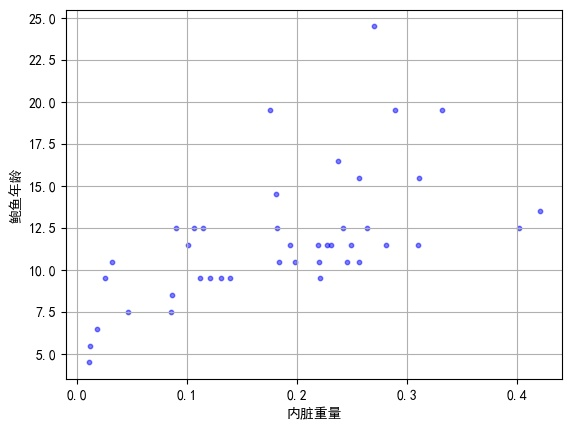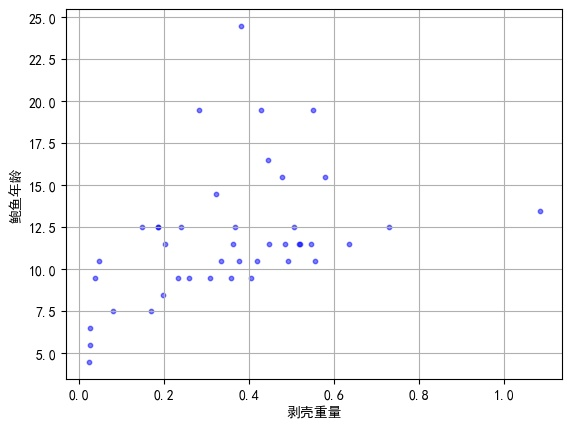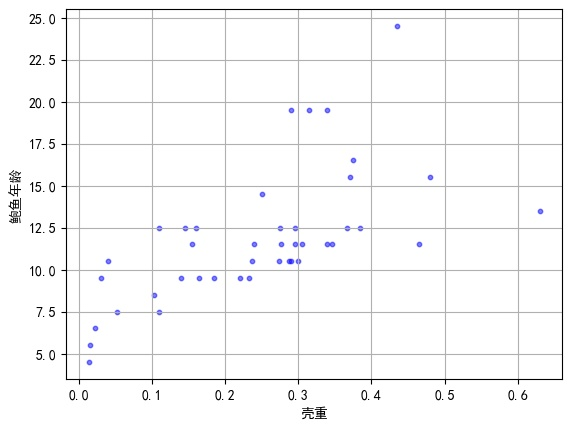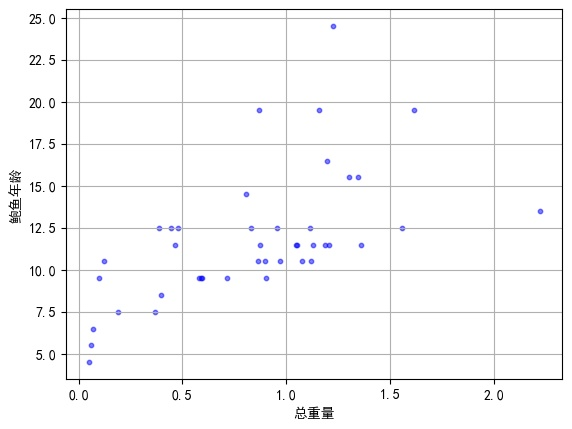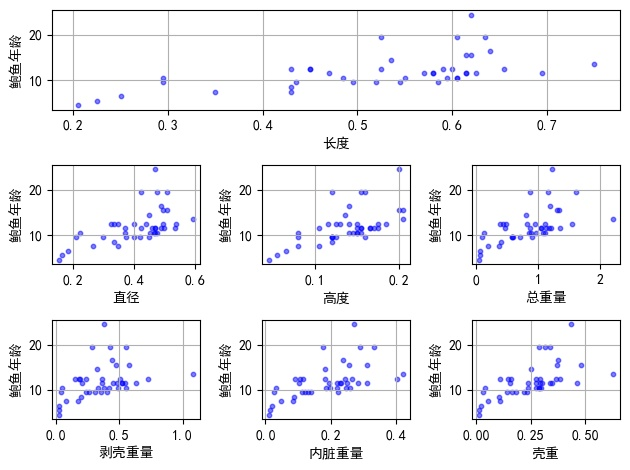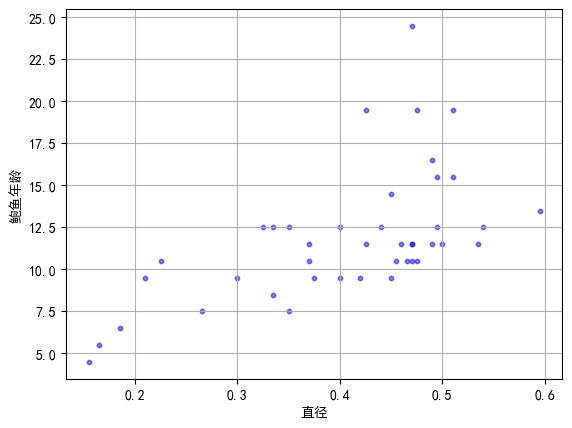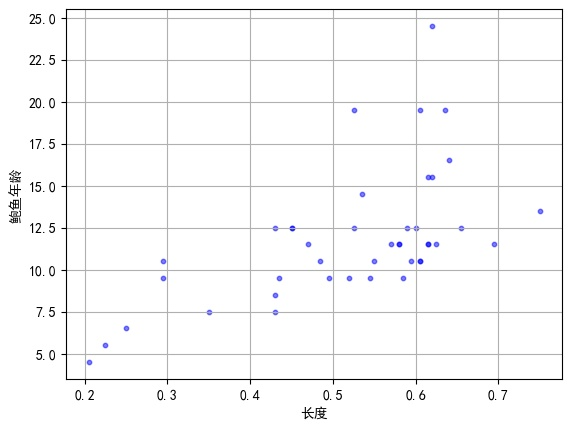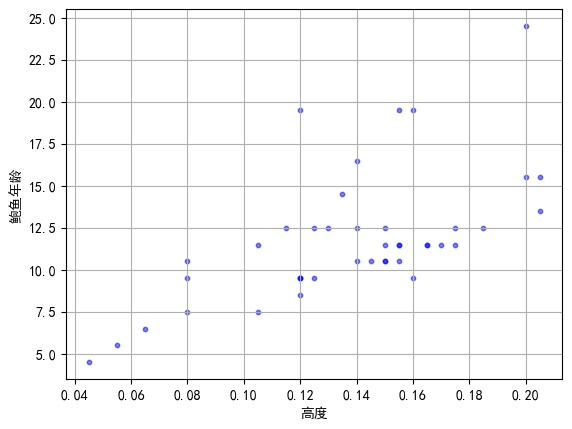展开全文sklearn 机器学习
• 线性回归虽然简单，但是容易出现问题：就是“欠拟合”和“过拟合”，欠拟合是由于我们并不能很好的拟合我们的训练数据，导致出现较大的训练误差；而过拟合是由于我们过度拟合训练数据，导致我们的模型过度复杂而产生...

线性回归虽然简单，但是容易出现问题：就是“欠拟合”和“过拟合”，欠拟合是由于我们并不能很好的拟合我们的训练数据，导致出现较大的训练误差；而过拟合是由于我们过度拟合训练数据，导致我们的模型过度复杂而产生较大的测试误差。

如下图所示：左边的图就是欠拟合，很明显我们用直线是无法很好的拟合训练数据的，最右边的就是产生了过拟合，中间的曲线就是比较好的。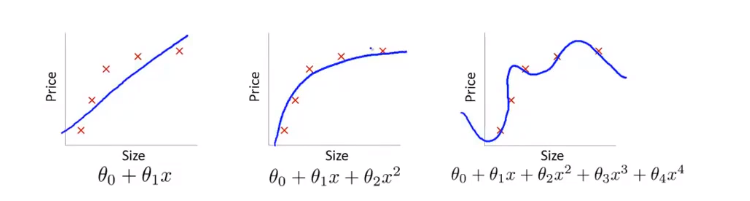解决欠拟合我们可以增加额外的特征，或者增加多项式（如 x 2 x^2 之类的）。

接下来介绍一种解决欠拟合的方法——局部加权线性回归

1.代价函数：
J ( θ ) = ∑ i = 1 m w ( i , i ) ( f ( x i ) − y i ) 2 J(\theta)=\sum_{i=1}^mw_{(i,i)}(f(x_i)-y_i)^2
其中 f ( x i ) f(x_i) 是我们的预测值， f ( x i ) = θ T x i f(x_i)=\theta^Tx_i w ( i , i ) w_{(i,i)} 是权重，它是通过要预测的点i与数据集中点的距离来确定的，距离越近， 权值越大，相应的误差值 ( f ( x i ) − y ) (f(x_i)-y) 影响就越大（可以达到增加多项式的效果），反之同理。我们通常使用如下的权重函数：
w ( i , i ) = e x p ( − ( x i − x ) 2 2 k 2 ) w_{(i,i)}=exp(-\frac{(x_i-x)^2}{2k^2})
其中，x是我们的要预测的点，k是我们需要指定的参数，他控制了权值随距离变化的速率。
接下来我们可以通过梯度下降或者正规方程的方法来求解我们的 θ \theta ，这里使用正规方程的解法，首先将我们的上面的代价函数写成矩阵的形式：
J ( θ ) = ( X θ − y ) T W ( X θ − y ) J(\theta)=(X\theta-y)^TW(X\theta-y)
其中X是我们的训练集，每一行是一个样本，y是我们的真实值，W是m大小的方阵，并且只有对角线上有值，其他地方都为0，（这里不懂得可以随便去一个样本大小为例子乘以下就知道了）。

最后我们就用对 J ( θ ) J(\theta) 关于 θ \theta 求导，并令其为0求出我们的 θ \theta 等于：
θ = ( X T W X ) − 1 X T W y \theta=(X^TWX)^{-1}X^TWy

2.代码实现：
这里直接用了机器学习实战上面的实现代码
2.1算法实现：

from numpy import *
def lwlr(testPoint,xArr,yArr,k=1.0):
xMat = mat(xArr); yMat = mat(yArr).T
m = shape(xMat)
weights = mat(eye((m)))  #创建一个单位矩阵
for j in range(m):
diffMat = testPoint - xMat[j,:]     #计算与数据集中其他点的距离
weights[j,j] = exp(diffMat*diffMat.T/(-2.0*k**2))  #计算权重
xTx = xMat.T * (weights * xMat)
if linalg.det(xTx) == 0.0:
print("This matrix is singular, cannot do inverse")
return
ws = xTx.I * (xMat.T * (weights * yMat))
return testPoint * ws

def lwlrTest(testArr,xArr,yArr,k=1.0):  #testArr是我们要预测的所有点的矩阵
m = shape(testArr)
yHat = zeros(m)
for i in range(m):
yHat[i] = lwlr(testArr[i],xArr,yArr,k)   #对于每一个要预测的点都要重新计算权值
return yHat


2.2绘制结果：

from numpy import *
import matplotlib.pyplot as plt
import regression

yHat = regression.lwlrTest(xArr,xArr,yArr,1.0)
xMat = mat(xArr)
srtInd = xMat[:,1].argsort(0)
xSort = xMat[srtInd][:,0,:]

fig = plt.figure()
ax.plot(xSort[:,1],yHat[srtInd])
ax.scatter(xMat[:,1].flatten().A, mat(yArr).T.flatten().A,s=2,c='red')
plt.show()


3.运行结果：
k=1.0（欠拟合）：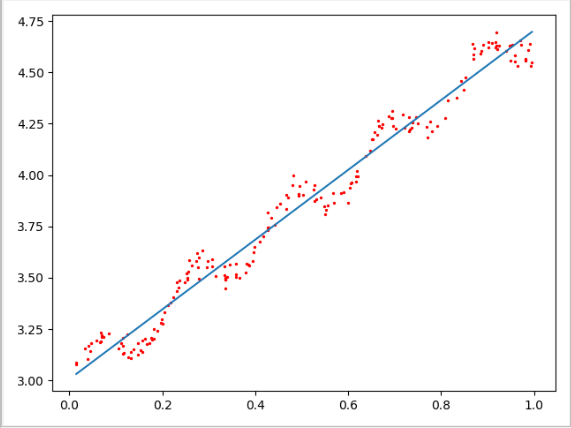k=0.01（拟合的比较好）：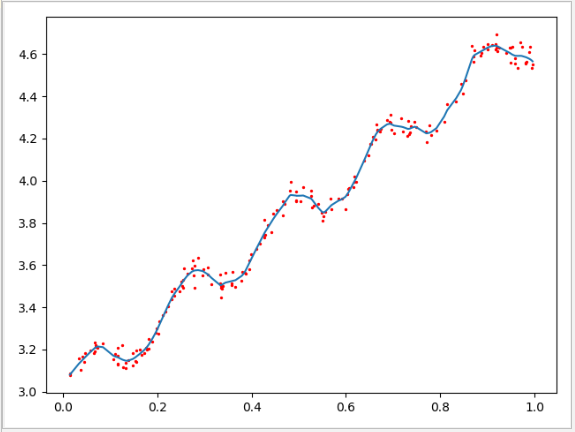k=0.003（过拟合）：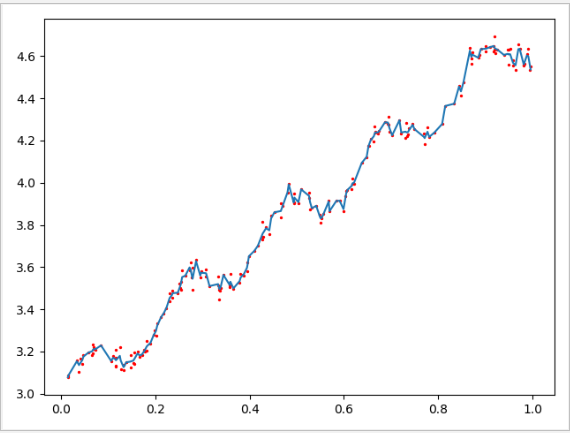在调用上面代码时，我们通过会选取不同的k值，以获得最佳效果。
通过上面我们也知道每预测一个点，我们都要重新计算权重W矩阵，增加了我们的计算量。

展开全文线性回归 欠拟合
• 数据下载地址：数据集下载 图像： 想要表示出这些数据背后...4)对应的系数，我们称它为回归系数，我们称这个方程为回归方程，我们称求出系数ωij的过程为回归。 只要我们能够通过某种方法求出了系数ω，我...线性回归 机器学习 人工智能
• 前面我们介绍了最基础的线性回归算法，也提到了它容易出现欠拟合的现象。那么，本文的局部加权线性回归将解决这个欠拟合问题。我觉得这个思路具有很好的迁移性，咱们一起好好看看。python 机器学习
• 文章描述了局部加权线性回归基本原理（英文），并且用MATLAB上实现了对一个机器人不规则路径的非线性拟合拟合。文档末尾附有代码。
• 但线性回归往往容易欠拟合，除了使用更复杂得函数拟合，还可以使用局部加权线性回归(locally weighted linear regression) 局部加权线性回归(LWLR) 在该算法中我们给待预测点附件得每个点赋予一定的权重，即在计算时...机器学习 python
• 局部加权线性回归算法(Local Weighted Linear Regression)及相关案例 大家好，我是W 这次讲线性回归，之前有说逻辑回归（Logistic Regression），虽然逻辑回归负责分类，可是在数据到达二值函数之前的逻辑都是线性...算法 python 机器学习
• ## 局部加权线性回归

千次阅读 2018-03-31 15:40:16
1、方法论 局部加权线性回归（Local Weights Linear Regression）也是一种线性回归，不同的是，普通线性回归是全局线性回归，使用全部的样本计算回归系数。而局部加权线性回归，通过引入权值（核函数），在预测的...
• 当要预测的样本序列不是明显的线性关系时，用线性回归会存在拟合不好的现象，即欠拟合。 局部加权回归定义： 以一个点 x为中心，向前后...线性回归局部加权回归的损失函数： 可以发现：局部加权回归对局部特征能较python 算法
• 前面博客有讲到，样本如果不是线性的可以通过多项式扩展，映射到多维空间来拟合。如此之外，还可以做一个局部加权线性回归（Locally Weighted Linear Regression，LWLR）。 ...
• 局部加权线性回归 local weighted linear regression 线性回归 用普通最小二乘法 Ordinary Least Square 即通过最小化误差的平方和寻找数据的最佳函数匹配 ∑ i = 1 m ( y − x i T w ) 2 \sum_{i=1}^{m}(y-x...机器学习
• 1. 前言： 我们知道线性回归的一个问题就是欠拟合，将不能取得很...其中一个非常有效的方法就是局部加权线性回归（LWLR）。2. 算法思想： 2.1. 比较线性回归： 2.2. 局部加权线性回归：（使用高斯核权重） ...机器学习 python
• 线性回归的缺点是容易出现欠拟合，采用局部加权线性回归，给与每一个待预测点附近的点一定的权重，在该子集上基于最小平方误差进行回归。这种算法每次预测前需要先选出相应数据子集。对应回归系数的解为： 其中W...
• 当然还是从最简单的线性回归开始，本文主要介绍无偏差的标准线性回归和有偏局部加权线性回归的理论基础以及相应的Python实现。标准线性回归标准线性回归的理论知识很简单，我们既可以写出它的标量表达式也可以写成...
• 线性回归 算法优缺点： 优点：结果易于理解，计算不复杂 缺点：对非线性数据拟合不好 适用数据类型：数值型和标称型 算法思想：   这里是采用了最小二乘法计算（证明比较冗长略去）。这种方式的优点是计算...python
• 欢迎关注”生信修炼手册”!对于回归而言，有线性模型和非线性模型两大模型，从名字中的线性和非线性也可以直观的看出其对应的使用场景，但是在实际分析中，线性模型作为最简单直观的模型，是我们分析...人工智能 python 机器学习 支持向量机
• 我们方法的新颖之处在于将局部加权线性回归模型应用于光谱反射率估计，并在CIELAB均匀色彩空间中使用高斯函数构造加权矩阵。 使用标准色表和一组纺织品样品，并通过数字RGB相机和十倍十倍的交叉验证，对提出的方法...研究论文
• 理解局部加权线性回归+源码实现 线性回归是在已有数据的基础上，通过建立含有未知参数的线性模型来拟合样本点。通过已有数据确定未知参数后，就可用回归模型来预测其他输入状态下的输出值。 一般地，线性回归分为...机器学习 python
• 一、线性回归（Linear Regression） 线性回归一般用来做预测，预测数值型的目标值，基本思想是有一个目标值的公式，然后依据数据，得到预测的目标值。 例如： 其中，称作回归系数，是输入特征，y为目标预测值。 ...机器学习 python 线性回归 笔记
• 这一篇文章，我们将首次介绍回归算法即对连续性的数据做出预测。回归一词的来历由来已久。“回归”是由英国著名生物学家兼统计学家高尔顿(Francis Galton,1822～1911.生物学家达尔文的表弟)在研究人类遗传问题时提...lr ridge...